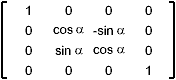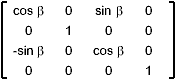# Rotations

Rotations in three-dimensions are considerably more complicated than two-dimensional rotations. In general, rotations are specified by a rotation axis and an angle. In two-dimensions there is only one choice of a rotation axis that leaves points in the plane.

### Right hand thumb rule

If the thumb points towards the positif axis (in the same direction as the vector that define the rotation) then positif rotations follow the other finger of the right hand.

A special case : Positive rotation directions about the coordinate axes are counterclockwise, when looking toward the origin from a positive coordinate position on each axis

## Coordinate-Axes Rotations

Around the x axis Around the y axis Around the z axis## How to get these matrix ? let's have a look first at the rotation around the z axis

 Web www.vrarchitect.net